Test: The PN - Junction

# Test: The PN - Junction

Test Description

## 20 Questions MCQ Test GATE Electrical Engineering (EE) 2023 Mock Test Series | Test: The PN - Junction

Test: The PN - Junction for Electronics and Communication Engineering (ECE) 2022 is part of GATE Electrical Engineering (EE) 2023 Mock Test Series preparation. The Test: The PN - Junction questions and answers have been prepared according to the Electronics and Communication Engineering (ECE) exam syllabus.The Test: The PN - Junction MCQs are made for Electronics and Communication Engineering (ECE) 2022 Exam. Find important definitions, questions, notes, meanings, examples, exercises, MCQs and online tests for Test: The PN - Junction below.
Solutions of Test: The PN - Junction questions in English are available as part of our GATE Electrical Engineering (EE) 2023 Mock Test Series for Electronics and Communication Engineering (ECE) & Test: The PN - Junction solutions in Hindi for GATE Electrical Engineering (EE) 2023 Mock Test Series course. Download more important topics, notes, lectures and mock test series for Electronics and Communication Engineering (ECE) Exam by signing up for free. Attempt Test: The PN - Junction | 20 questions in 60 minutes | Mock test for Electronics and Communication Engineering (ECE) preparation | Free important questions MCQ to study GATE Electrical Engineering (EE) 2023 Mock Test Series for Electronics and Communication Engineering (ECE) Exam | Download free PDF with solutions
 1 Crore+ students have signed up on EduRev. Have you?
Test: The PN - Junction - Question 1

### What is the effect of cascading the amplifier stages?​

Detailed Solution for Test: The PN - Junction - Question 1

Due to cascading voltage gain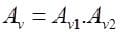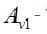Voltage gain of 1st satge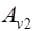- Voltage gain of 2nd stage
So it increases.
While upper cutoff frequency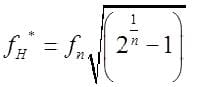While lower cutoff frequency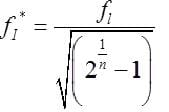Increases compare to single state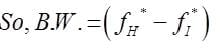As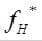decreases and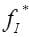increases.

Test: The PN - Junction - Question 2

### Generally, the gain of a transistor amplifier falls at high frequencies due to the​

Detailed Solution for Test: The PN - Junction - Question 2

The gain of a transistor falls at high frequencies due to the various parasitic capacitances of the devices.

Test: The PN - Junction - Question 3

### For the MOSFET shown in the figure, the threshold voltage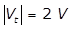and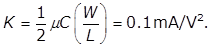The value of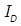(in mA) is ___________.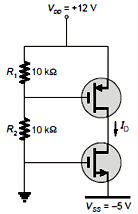Detailed Solution for Test: The PN - Junction - Question 3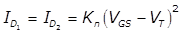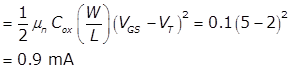Test: The PN - Junction - Question 4

The trans conductance gm of the transistor shown in figure is 10 mS. The value of the input resistance RIN   seen by source is -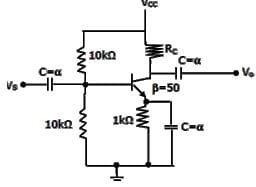Detailed Solution for Test: The PN - Junction - Question 4

Input Resistance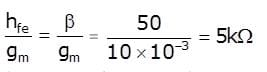But overall input resistance seen from source is
RIN = 10 || 10|| 5
RIN = 2.5 kΩ.

Test: The PN - Junction - Question 5

In the circuit shown in figure (a), if the input is given as in figure (b). then the output will be (Assume that the time constant is very large)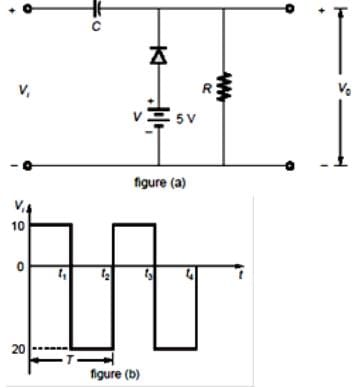Detailed Solution for Test: The PN - Junction - Question 5

The analysis will begin with the period t1-t2 of the input signal since the diode is in its short circuit state.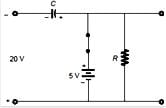The output is across R, but it is also across 5 V battery so V0 = 5V
Applying KVL around the input loop
-20-5 + Vc = 0
Vc= 25 V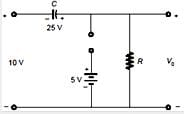the capacitor will therefore charge up to 25 V from t2 to t3 the circuit will appear as shown below by applying KVL in the outside loop,
-10-25+V0 =0
V0 = 35 V
∴ The output waveform will be as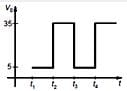Test: The PN - Junction - Question 6

The input resistance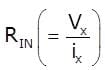of the circuit in figure is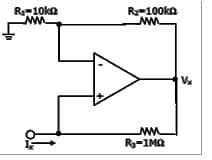Detailed Solution for Test: The PN - Junction - Question 6

Here Ix =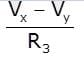But Vy =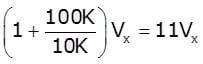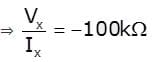Vy = 11 Vx
Ix = (Vx - Vy) / (1M Ω)
= (Vx - 11 Vx) / (106 Ω)
= -10 Vx/ 106
Therefore V/ Ix = 106/ (-10) = -100 K Ω

Test: The PN - Junction - Question 7

The following circuit has R = 10kΩ, C = 10μF. The input voltage is a sinusoid at 50Hz with an rms value of 10V. Under ideal conditions, the current is from the source is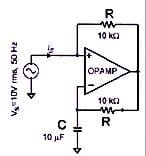Detailed Solution for Test: The PN - Junction - Question 7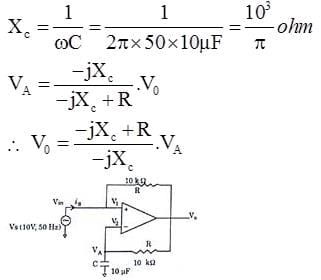Assuming virtual ground,
Vm = VA = 10V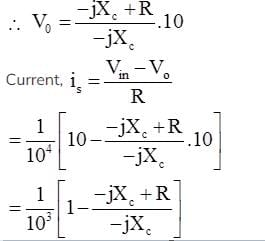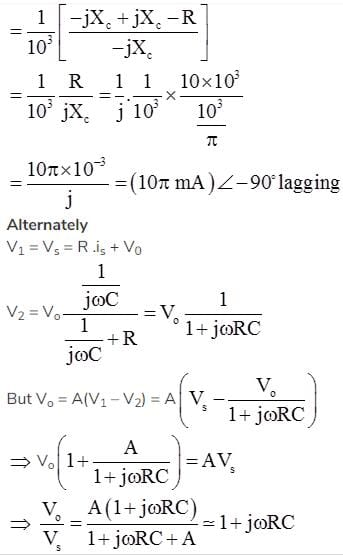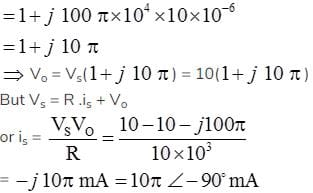Test: The PN - Junction - Question 8

For the given n-channel MOSFET, determine the % decrease in the drain current at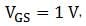if the n-channel between the two n-doped regions is removed. Given,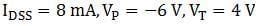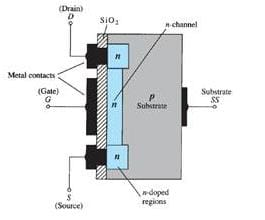Detailed Solution for Test: The PN - Junction - Question 8

Given is a depletion-type MOSFET. The drain current is given as,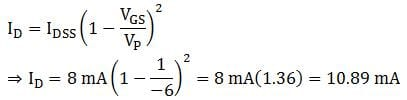When the n-channel between the two-doped regions is removed, it resembles an enhancement-type MOSFET as shown below.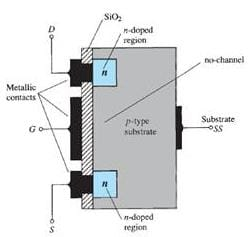For an enhancement-type MOSFET,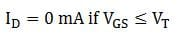Since,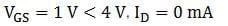Thus, there is a 100% decrease in the drain current.

Test: The PN - Junction - Question 9

The feedback used in the circuit shown in figure can be classified as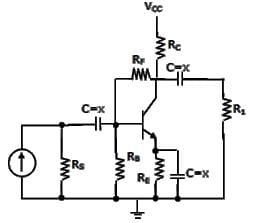Detailed Solution for Test: The PN - Junction - Question 9

Equivalent circuit can be drawn with input voltage comparison and current feedback. It is shunt-shunt feedback.

Test: The PN - Junction - Question 10

For the given network, determine the output waveform. The circuit components are given below: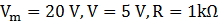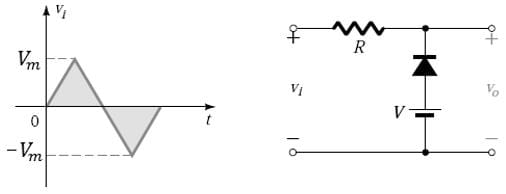Detailed Solution for Test: The PN - Junction - Question 10

The output voltage is across the series combination of 5 V supply, and the diode.
In the positive region of the input signal, the diode is an open circuit. The voltage drop across the resistor is zero. Hence,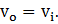There is a change in state at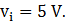Hence, the output is fixed at 5 V until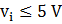, and above that the output waveform is same as the input waveform.
In the negative region of the input signal, the diode is on. When the diode is short-circuited, the output voltage is directly across the 5 V supply, and hence, the output is fixed at 5 V.
Hence, the output waveform is obtained as: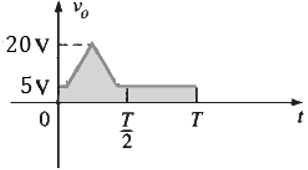Test: The PN - Junction - Question 11

In the voltage doubler circuit shown in the figure, the switch ‘S’ is closed at t = 0. Assuming diodes D1 and D2 to be ideal, load resistance to be infinite and initial capacitor voltages to be zero, the steady state voltage across capacitors C1 and C2 will be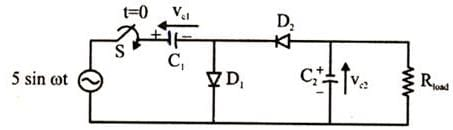Detailed Solution for Test: The PN - Junction - Question 11

Since RLOAD is infinite, we can replace with open circuit
C1 will be charged in first +Ve half cycle
VC1 = +5v
C2 will be charged in first –Ve half cycle
VC2 = -10v

Test: The PN - Junction - Question 12

A clipper circuit is shown below.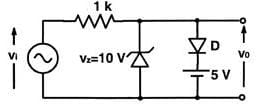Q. which of the following is the transfer characteristics of above circuit?

Detailed Solution for Test: The PN - Junction - Question 12

When –0.7 V < Vi < 5.7 V output will following put, because zener diode and normal diodes are off
When Vi < –0.7 V Zener diode forward bias and V0 = –0.7 V
When Vi > 5.7 V Diode is forward bias and Vn = 5.7 V

Test: The PN - Junction - Question 13

Including a small resistance in the source (emitter) of a CS (CE) amplifier provides the designer with a tool to improve the performance, that is

Detailed Solution for Test: The PN - Junction - Question 13

If there is a small resistance added in the emitter of CE configuration will effect the gain as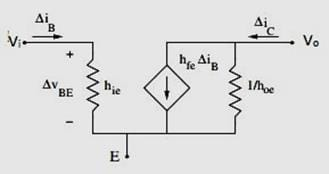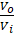=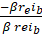=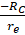=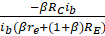In the second case, the gain decreased due to emitter resistance RE. as gain decreases , to maintain gain bandwidth product constant, bandwidth increases.

Test: The PN - Junction - Question 14

The transistor in the given circuit should always be in the active region.Take VcE(sat)=0.2V, VEE= 0.7 V The maximum value of Rc in Ω which can be used is __________.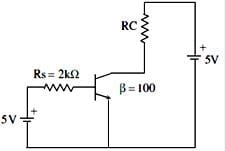Detailed Solution for Test: The PN - Junction - Question 14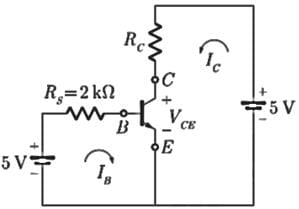Given: VCE(sat) = 0.2
VBE = 0.7
β = 100
Writing KVL around the input loop
5 - IBRS - 0.7 = 0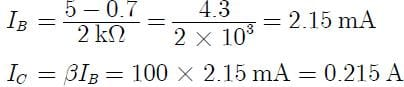Writing KVL around the output loop
5 - ICRC - VCE = 0
VCE = 5 - (0.215)RC
For active region VCE > VCE(sat)
or,      VCE > 0.2 V
So,     5 - 0.215RC > 0.2
0.215RC < 5 - 0.2
Hence RC(min) = 4.8 / (0.215) = 22.32 Ω

Test: The PN - Junction - Question 15

In the circuit shown , function of diode D1 is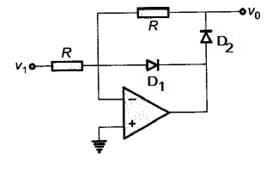Detailed Solution for Test: The PN - Junction - Question 15

If D1 would have not been there then for V1<0, D2 ON and op-amp would have been in open loop mode.
In general Op-amp has high open loop gain which may cause the saturation.
By adding D1 saturation is avoided.

*Answer can only contain numeric values
Test: The PN - Junction - Question 16

For the network of figure determine the input impedance Zi (in kΩ )Assume Vcc=22V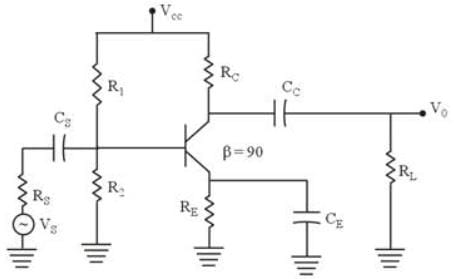RS = 10 kΩ, R1 = 56 kΩ, R2 = 8.2 kΩ
CE = 20 μF, RE = 1.5 kΩ
RC = 6.8 kΩ, CC = 10 μF

Detailed Solution for Test: The PN - Junction - Question 16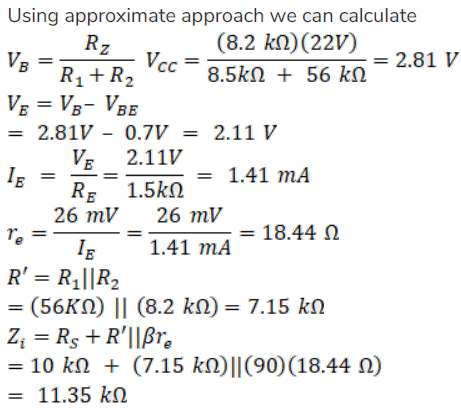*Answer can only contain numeric values
Test: The PN - Junction - Question 17

For the common Emitter configuration circuit shown below,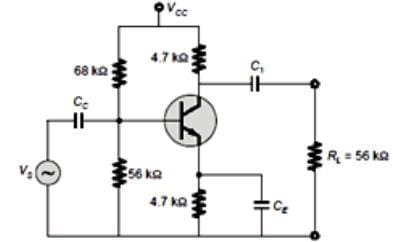If Ic = 1.5mA then the magnitude of voltage gain is __________.

Detailed Solution for Test: The PN - Junction - Question 17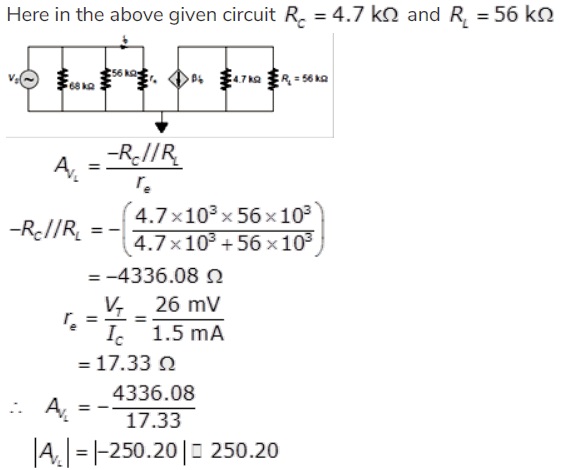Test: The PN - Junction - Question 18

For the given fixed-bias configuration, determine the parameters re & ro for its equivalent re  model of the transistor, if the input impedance of the network is 1 kΩ and the output impedance is 4 kΩ.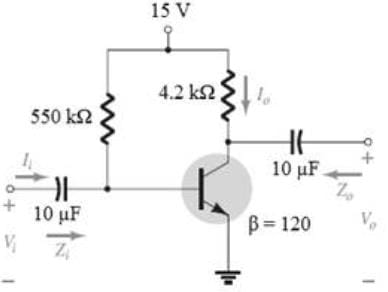Detailed Solution for Test: The PN - Junction - Question 18

The ac equivalent circuit for the network is given below.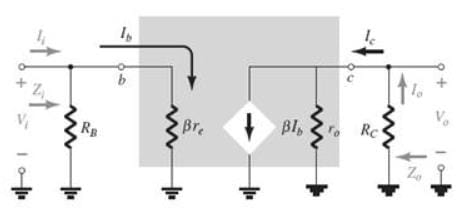The input impedance of the network is given as,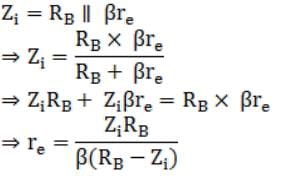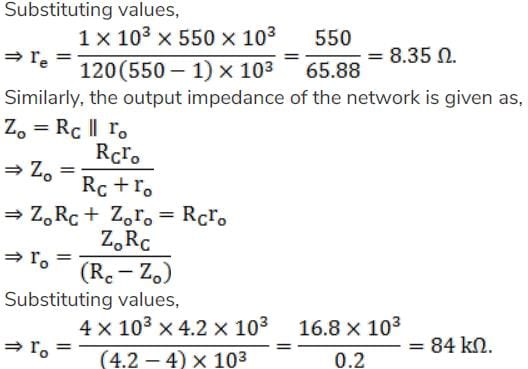Test: The PN - Junction - Question 19

Identify the type of connection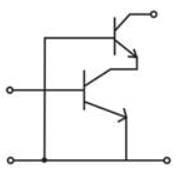Test: The PN - Junction - Question 20

When the controlling voltage of an n-channel JFET is kept at 0 V, and a voltage greater than the pinch-off voltage is applied between the drain and the source terminal, the JFET:

Detailed Solution for Test: The PN - Junction - Question 20

The transfer characteristics for an n-channel JFET when the controlling voltage, VGS = 0V  is shown below.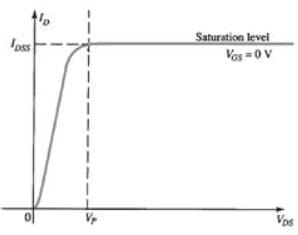It can be seen from the characteristics that when the voltage between the drain and source (VDS) becomes greater than the pinch-off voltage (VP), the drain current remains essentially the same, as the region between the two depletion regions will increase in length. The drain current ID is fixed at the value ID = IDSS, where IDSS is the maximum drain current.
Hence, the JFET acts as a current source as shown below, for VGS = 0V, VDS>Vp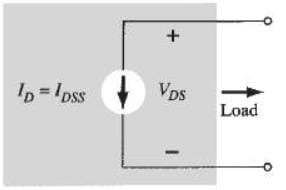## GATE Electrical Engineering (EE) 2023 Mock Test Series

18 docs|208 tests
 Use Code STAYHOME200 and get INR 200 additional OFF Use Coupon Code
Information about Test: The PN - Junction Page
In this test you can find the Exam questions for Test: The PN - Junction solved & explained in the simplest way possible. Besides giving Questions and answers for Test: The PN - Junction, EduRev gives you an ample number of Online tests for practice

## GATE Electrical Engineering (EE) 2023 Mock Test Series

18 docs|208 tests